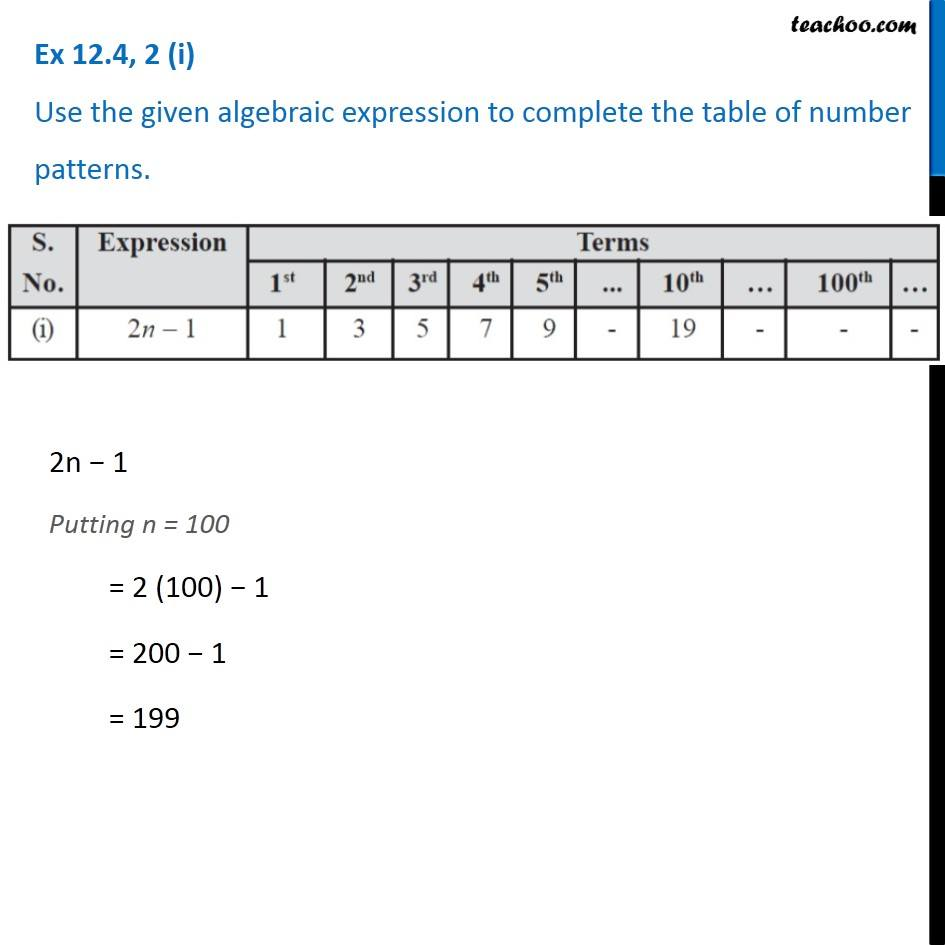1. Chapter 12 Class 7 Algebraic Expressions
2. Concept wise
3. Number patterns

Transcript

Ex 12.4, 2 (i) Use the given algebraic expression to complete the table of number patterns. 2n − 1 Putting n = 100 = 2 (100) − 1 = 200 − 1 = 199